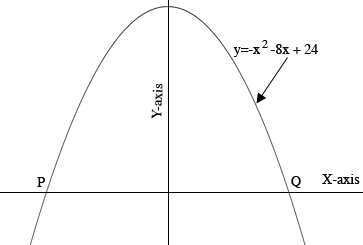SEARCH HOMEMath Central Quandaries & QueriesQuestion from Malen: A hotel entrance makes a parabolic arch that can be represented by the quadratic function, y= -x^2-8x+24, where y is the height of the arch and x is the distance from wall to wall in the feet. What is the distance between the two walls of the arch.Hi Malen,

Draw a diagram!What are the coordinates of $P?$ What are the coordinates of $Q?$ What is the distance between $P$ and $Q?$

HarleyMath Central is supported by the University of Regina and The Pacific Institute for the Mathematical Sciences.